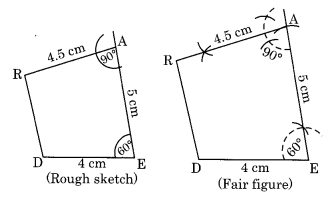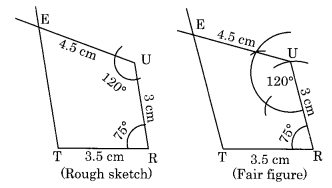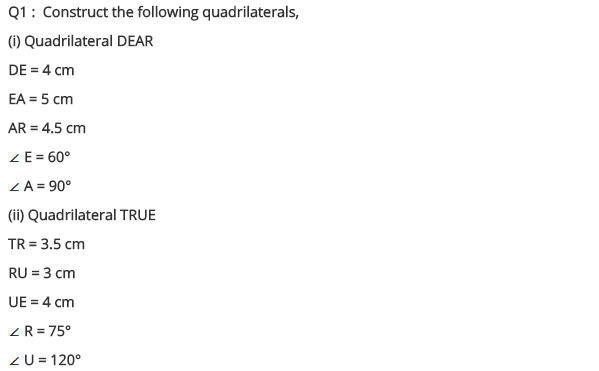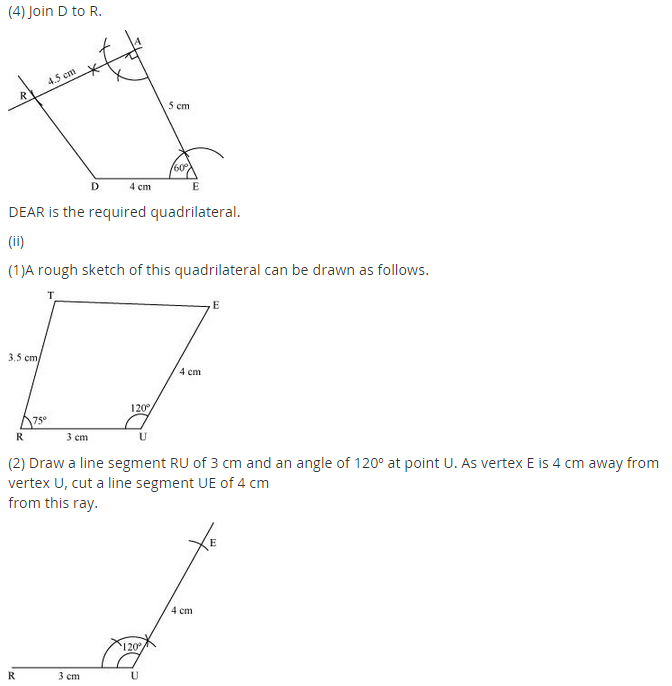# NCERT Solutions for Class 8 Maths Chapter 4 Practical Geometry Ex 4.4

NCERT Solutions for Class 8 Maths Chapter 4 Practical Geometry Ex 4.4

### NCERT Solutions for Class 8 Maths Chapter 4 Practical Geometry Exercise 4.4

Ex 4.4 Class 8 Maths Question 1.
DE = 4 cm, EA = 5 cm, AR = 4.5 cm, ∠E = 60°, ∠A = 90°
TR = 3.5 cm, RU = 3 cm, UE = 4.5 cm, ∠R = 75°, ∠U = 120°
Solution:
(i) Construction:Step I: Draw DE = 4 cm.
Step II: Draw an angle of 60° at E.
Step III: Draw an arc with centre E and radius 5 cm to meet the angle line at A.
Step IV: Draw an angle of 90° at A and cut AR = 4.5 cm.
Step V: Join DR.
Thus, DEAR is the required quadrilateral.

(ii) Construction:Step I: Draw TR = 3.5 cm
Step II: Draw an angle of 75° at R and cut RU = 3 cm.
Step III: Draw an angle of 120° at U and cut UE = 4.5 cm.
Step IV: Join TE.
Thus, TRUE is the required quadrilateral.## SabDekho

The Complete Educational Website